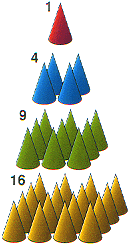# Squares

The first ten square numbers are –
1, 4, 9, 16, 25, 36, 49, 64, 81, 100 ...

Each is the result of multiplying a number by itself –
1 × 1, 2 × 2, 3 × 3, 4 × 4, 5 × 5 ...
– which can also be written –
12, 22, 32, 42, 52 ...
– where the small 2 means 'squared'.

The square of 7 is 49, and working backwards, we say the square root of 49 is 7.

All square numbers end in either a 0, 1, 4, 5, 6 or a 9. So if someone were to ask you if 225,736,192 was a square number, you could say 'no' right away because it ends in a 2.

If you subtract a square number from the next square number you get the odd numbers 3, 5, 7, 9 ...The first four square numbers

Based on the book Numbers: Facts, Figures & Fiction.